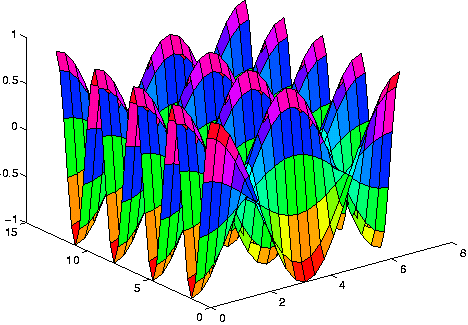# MATLAB

(redirected from Matlab programming language)

## MATLAB

(mathematics, language, application)
A high-level language and interactive program from The MathWorks for numeric computation and visualisation. MATLAB supports numerical analysis, matrix computation, signal processing, linear algebra, statistics, Fourier analysis, filtering, optimisation and numerical integration. It can output two and three dimensional graphics and can be integrated with C, C++, Fortran, Java, COM and Microsoft Excel.

http://mathworks.com/products/matlab/.

## MATLAB

(MATrix LABoratory) A programming language for technical computing from The MathWorks, Natick, MA (www.mathworks.com). Used for a wide variety of scientific and engineering calculations, especially for automatic control and signal processing, MATLAB runs on Windows, Mac and a variety of Unix-based systems. Developed by Cleve Moler in the late 1970s and based on the original LINPACK and EISPACK FORTRAN libraries, it was initially used for factoring matrices and solving linear equations. Moler commercialized the product with two colleagues in 1984. MATLAB is also noted for its extensive graphics capabilities. The following MATLAB commands generate the 3D graph below:

```     x=(0:2*pi/20:2*pi)';
y=(0:4*pi/40:4*pi)';
[X,Y] = meshgrid(x,y);
z= cos(X).*cos(2*Y);
surf (x,y,z);
```

The Resulting GraphThis graph was computed from the lines of code above and is an example that might be used in multivariate calculus. (Image courtesy of Dr. Mark S. Gockenbach, Michigan Technological University, www.math.mtu.edu/~msgocken/intro/intro.html)
Mentioned in ?
References in periodicals archive ?
In addition, the original FORTRAN source code has now been converted into the widely used MATLAB programming language.
They use the Matlab programming language and toolboxes, as well as exercises, to give readers a hands-on method to learn the concepts.
Matlab's functions are easily completed by a user through the Matlab programming language.
This classroom-tested text provides comprehensive coverage of the MATLAB programming language used by engineers.
This comprehensive software package is based on the Matlab programming language and tool set and allows the evaluation of numerous effects on algorithm performance.
A pedagogical presentation containing over 150 problems and programming exercises, and numerous figures and diagrams, and code fragments in the MATLAB programming language helps the reader with limited experience of numerical methods reach an understanding of this subject.
When combined with the long-established productivity gain of working in the intuitive MATLAB programming language, the accelerated MATLAB performance enables users to skip the additional step and trouble of recoding their work in C.

Site: Follow: Share:
Open / Close